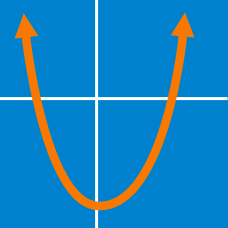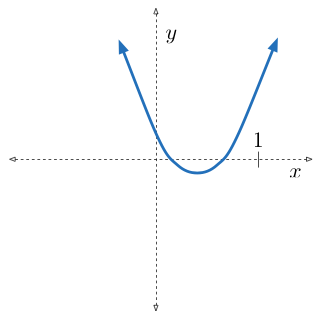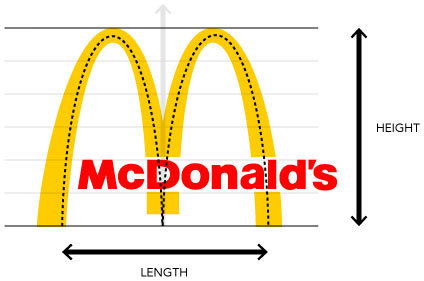Algebra

Challenge Quizzes

If $\alpha,\beta$ are the roots of the quadratic equation

$x^2-\left( 3+2^{\sqrt{\log_23}}-3^{\sqrt{\log_32}} \right)x-2\left( 3^{\log_32}-2^{\log_23} \right) =0$,

then find the value of $\left( \alpha^2+\alpha\beta+\beta^2 \right)$.

Find the number of quadratic equations which are unchanged by squaring their roots.Positive integers $a, b$ and $c$ satisfy the condition that the quadratic equation $ax^2 - bx +c =0$ has 2 distinct real roots that are strictly between 0 and 1. What is the minimum value of $a$?

How many integral values of $a$ lie in the interval $[-1000,1000]$ for which the inequality ${25}^x+(a+2).5^x-(a+3)<0$ is satisfied for at least one real $x$.If the McDonald's logo were stored as a set of pixels, enlargement would quickly result in distorted or pixelated images, which are an eyesore. As such, companies often make vector images of their logos, in which the information is stored as mathematical formulae. Such vector images are easily scaled while maintaining sharp, crisp images.

As a first approximation, the logo is deconstructed and approximated as 2 parabolic curves of the form $y = -A(x-5)^2$ and $y = - A (x+5)^2$. The McDonald's logo has a height to length ratio of 1.05. What is $A$?

×